Thursday 01st October 2020CBSE Guess > Papers > Important Questions > Class XII > 2009 > Maths > Mathematics By Anil Kumar Tondak

CBSE CLASS XII

Matrices

Q. 1. Construct a 3´4 matrix, whose elements are given by aij=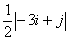Q. 2. If A =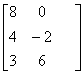and B =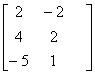, then find the matrix X, such that 2A + 3X = 5B.

Q. 3. If A =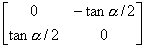and I =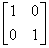, then show that I+A= (I – A)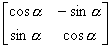Q. 4. Express the matrix A=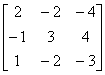as the sum of symmetric and skew-symmetric matrix
Q. 5. Obtain the inverse of the matrix A =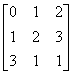using elementary transformations.

Q. 6. If f(x)=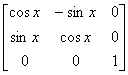Prove that f(x). f(y) = f(x + y)

Q. 7. Show that the matrix B¢AB is symmetric or skew-symmetric according as A is symmetric or skew symmetric.

Q. 8. If A and B are invertible matrices of the same order, then prove that (AB)-1 = B-1A-1
Q. 9. Let f(x) = x2 – 5x + 6. Find f(A) If  A =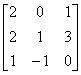Q. 10. If A =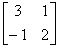Show that A2 -5A + 7I = 0, Use this to find A4.
Q. 11. Express the matrix A =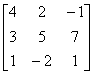as the sum of a symmetric and a skew-symmetric matrix.
Q. 12. Find the values of x, y, z if the matrix A =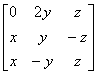satisfy the equation A¢A = I3.

Q. 13. Show that: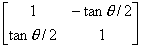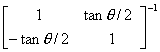=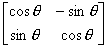Q. 14. Show that the following system of equations is consistent 2x – y + 3z = 5, 3x + 2y – z = 7, 4x + 5y – 5z = 9 Also, find the solution.

Prepared By Anil Kumar Tondak
M.Sc.(Maths), M.Ed., M.A.(Eco),M.B.A.(Mkt)
Mob No. 9811363962

 Maths